#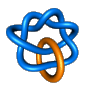Knot theory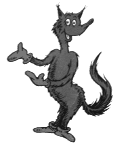Note: This page is part of the KnotPlot Site, where you'll find many more pictures of knots and links as well as MPEG animations and lots of things to download.
Knot theory is a branch of algebraic topology where one studies what is known as the placement problem, or the embedding of one topological space into another. The simplest form of knot theory involves the embedding of the unit circle into three-dimensional space. For the purposes of this document a knot is defined to be a closed piecewise linear curve in three-dimensional Euclidean space R3. Two or more knots together are called a link. Thus a mathematical knot is somewhat different from the usual idea of a knot, that is, a piece of string with free ends. The knots studied in knot theory are (almost) always considered to be closed loops.

Two knots or links are considered equivalent if one can be smoothly deformed into the other, or equivalently, if there exists a homeomorphism on R3 which maps the image of the first knot onto the second. Cutting the knot or allowing it to pass through itself are not permitted. In general it is very difficult problem to decide if two given knots are equivalent, and much of knot theory is devoted to developing techniques to aid in answering this question. Knots that are equivalent to polygonal paths in three-dimensional space are called tame. All other knots are known as wild. Most of knot theory concerns only tame knots, and these are the only knots examined here. Knots that are equivalent to the unit circle are considered to be unknotted or trivial.

The simplest non-trivial knot is the trefoil knot which comes in a left and a right handed form.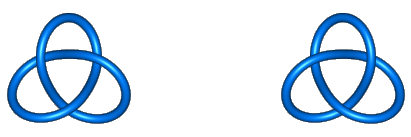It is not too difficult to see (but slightly more difficult to prove) that the trefoil is not equivalent to the unknot. Also, the right and left handed versions of the trefoil are only equivalent if the homeomorphism mapping one into the other includes a reflection (other knots, such as the Figure-8 knot are equivalent to their mirror images, these knots are known as achiral knots).

## Knot tables

Help keep the KnotPlot Project alive! Click on the button below to make a donation.

Use your credit card or PayPal account (PayPal account not needed).

Knots have been catalogued in order of increasing complexity. One measure of complexity that is often used is the crossing number, or the number of double points in the simplest planar projection of the knot. There is only one knot with crossing number three (ignoring mirror reflections), the trefoil or cloverleaf knot. The figure-8 knot is the only knot with a crossing number of four. There are two knots with a crossing number of five, three with a crossing number of six, and seven knots with a crossing number of seven. From there on the numbers increase dramatically. There are 12,965 knots with 13 or fewer crossings in a minimal projection and 1,701,935 with 16 or fewer crossings. Following are pictures of the sixteen simplest knots: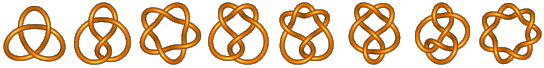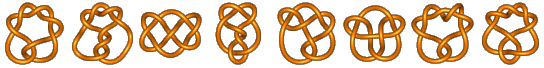Knots such as the square knot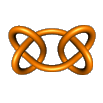are usually excluded from knot tables because they can be constructed of simpler knots. Knots that cannot be split into two or more simpler knots are called prime.

It is not always easy to tell when a given picture of a knot is the simplest possible picture for that knot. For example the following is the unknot: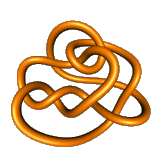=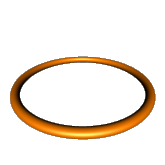?

The interested reader may enjoy trying to untangle this beast by making a sequence of knot diagrams. If you give up, look at MPEG animation of the untangling (106 kbytes). This unknot image is also available in raycasted and raytraced 608x608 pixel JPEG versions (about 130 kbytes each).

## KnotPlot Relaxations

In the first three of the following animations, the starting configuration of the knot is specified by the Conway notation. The knot then relaxes under the simple dynamics used by KnotPlot (the arrows are force vectors).

## Braid theory

See the companion page on braid theory (incomplete).

## Higher dimensional knot-theory

Knot theory is extendible into higher dimensions. Generalized knot theory considers embeddings of the (N - 2)-dimensional sphere into an N dimensional sphere. In 4D knot theory we consider the embeddings of 2-spheres. There are a number of ways to construct knotted 2-spheres in 4D.
• Suspended knots --- A ordinary knot in 3D can be suspended in 4D to create a knotted 2-sphere.
• Spun knots --- One problem with suspended knots is that they are not smooth at the poles. While this might not seem like an important fact, it is actually not possible in general to smooth out the poles. One way to get a smoothly embedded sphere in 4D is to spin a 3D knot about a plane in 4D.
• Twist-spun knots --- A generalization due to Zeeman of spinning. This method produces knot types that that cannot be produced by ordinary spinning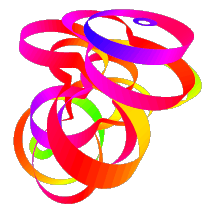Projection of a spun knot in 4d with transparent bands on the surface. Look at an MPEG animation the same knot rotating rigidly in 4d (418 kbytes).

Some strange things happen in higher dimensions with links, see the Linking Spheres page.